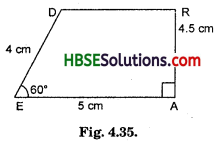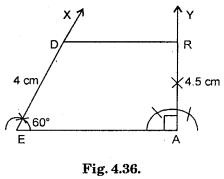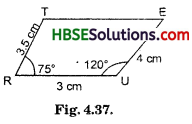# HBSE 8th Class Maths Solutions Chapter 4 Practical Geometry Ex 4.4

Haryana State Board HBSE 8th Class Maths Solutions Chapter 4 Practical Geometry Ex 4.4 Textbook Exercise Questions and Answers.

## Haryana Board 8th Class Maths Solutions Chapter 4 Practical Geometry Exercise 4.4

Question 1.
DEAR
DE = 4 cm
EA = 5 cm
AR = 4.5 cm
∠E = 60°
∠A = 90°
TRUE
TR = 3.5 cm
RU = 3 cm
UE = 4 cm
∠R = 75°
∠U = 120°
Solution:
Rough Sketch :Steps of Construction :
(i) Draw EA = 5 cm.
(ii) Make ∠DEA = 60° and ∠EAR = 90°.(iii) Cut off RA = 4.5 cm and DE = 4 cm.
(iv) Join DR.
(v) DEAR is the required quadrilateral.(ii) Rough SketchSteps of Construction :
(i) Draw RU = 3 cm.
(ii) Construct ∠XRU = 75° and ∠RUY = 120°.
(iii) Cut off RT = 3.5 cm and UE = 4 cm.
(iv) Join TE.
(v) TRUE is the required quadrilateral.